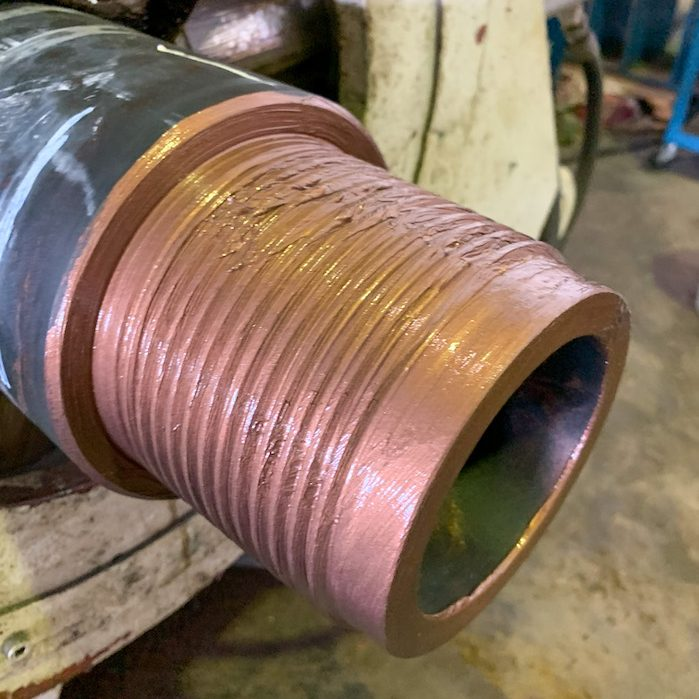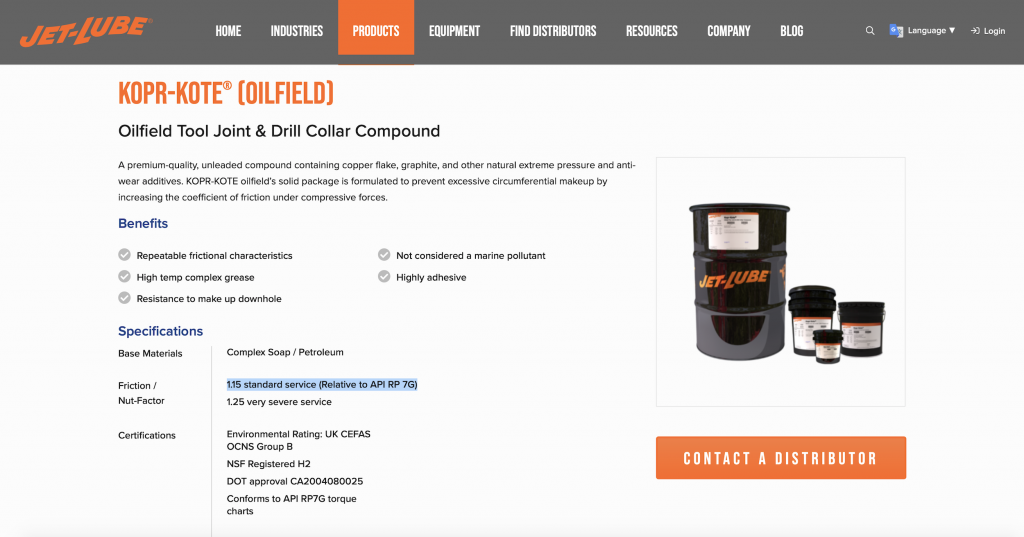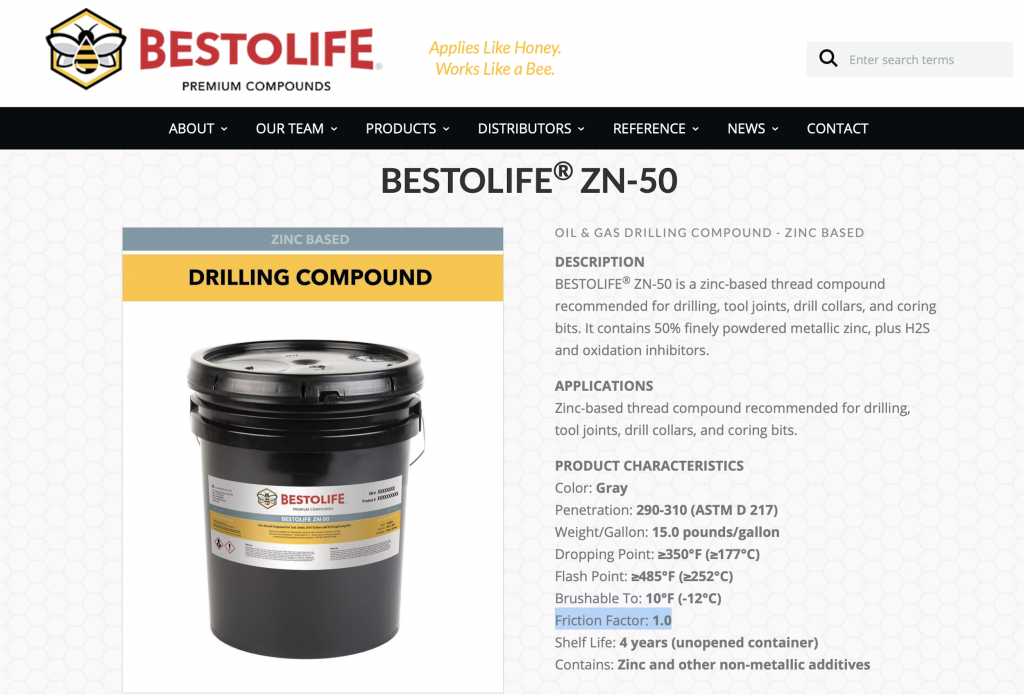### DPM-FAQSeries Topic 1: Thread Compound Friction Factor

1. What is thread compound (dope) friction factor and how to calculate Make-Up Torque (MUT) for different friction factors?

The thread compound friction factor helps to determine the correct amount of make-up torque (MUT) required for rotary-shouldered connections. The MUT calculations for rotary-shouldered connections in API 7G were based on zinc or lead-based thread compounds, which have a friction factor of 1.0. This means no adjustment needs to be made to the MUT. The compound manufacturer will typically specify the friction factor on the website and in the datasheet.Figure 1: KOPR-KOTE by JET-LUBE has Friction Factor of 1.15 – 1.25.Figure 2: ZN50 by BESTOLIFE has Friction Factor of 1.0.

The MUT should be multiplied by the friction factor for adjustment. So for a 30,000 ft MUT requirement with a dope of friction factor 1.15, the actual MUT should be 30,000 x 1.15 = 34,500 ft lbs. If the same MUT was required and the dope had a friction factor 0.8, then the actual MUT should be 30,000 x 0.8 = 24,000 ft lbs.

Top